## Poll

 I love math!21 votes (45.65%) Math is great.14 votes (30.43%) My religion is mathology.6 votes (13.04%) Women didn't speak to me until I was 30.3 votes (6.52%) Total eclipse reminder -- 04/08/202412 votes (26.08%) I steal cutlery from restaurants.3 votes (6.52%) I should just say what's on my mind.6 votes (13.04%) Who makes up these awful names for pandas?5 votes (10.86%) I like to touch my face.12 votes (26.08%) Pork chops and apple sauce.10 votes (21.73%)

46 members have voted

ThatDonGuyJoined: Jun 22, 2011
• Posts: 5956
June 24th, 2020 at 3:49:18 PM permalink
Quote: unJon

Quote: ThatDonGuy

Quote: charliepatrick

Is this the same as tossing six coins and seeing whether you get three or more heads?

The solution is to consider combinations and the 32 possibilities. Ranging from 0 thru 6 they are 1 6 15 20 15 6 1. So the chances of 3-3 is 20/64 and a strict majority 22/64. So the total chances are 42/64 = 65.625%.

it may be the same as tossing two red coins, two white coins, and two blue coins, and seeing if at least one coin of each color comes up heads

Why?
I don�t see anywhere in the problem that would stop me from using bullet # 3 on zombie #1 if the first two shots missed zombie #1.

Again, it depends on whether you have time to determine the target of each shot after seeing the results of the previous shots.

If you do not, then the best strategy is to shoot #1 twice, #2 twice, and #3 twice; you have a 75% chance of killing each one separately, so the probability of killing all three is 27/64.

If you do have time, then the best strategy is along the lines of, shoot at #1 until killed (although you can't really "kill" a zombie, now can you?), then shoot at #2 until killed, then shoot at #3.
The possible results, where Y is a successful shot and N an unsuccessful one, are:
No misses: (1/2)3 = 1/8
One miss: the miss can be any of the first three shots, so it's C(3,1) x (1/2)4 = 3/16
Two misses: the two misses can be any of the first four shots, so it's C(4,2) x (1/2)5 = 3/16
Three misses: the three misses can be any of the first five shots, so it's C(5,3) x (1/2)6 = 5/32
The sum = 21/32

unJonJoined: Jul 1, 2018
• Posts: 4352
June 24th, 2020 at 4:29:01 PM permalink
Quote: ThatDonGuy

Quote: unJon

Quote: ThatDonGuy

Quote: charliepatrick

Is this the same as tossing six coins and seeing whether you get three or more heads?

The solution is to consider combinations and the 32 possibilities. Ranging from 0 thru 6 they are 1 6 15 20 15 6 1. So the chances of 3-3 is 20/64 and a strict majority 22/64. So the total chances are 42/64 = 65.625%.

it may be the same as tossing two red coins, two white coins, and two blue coins, and seeing if at least one coin of each color comes up heads

Why?
I don�t see anywhere in the problem that would stop me from using bullet # 3 on zombie #1 if the first two shots missed zombie #1.

Again, it depends on whether you have time to determine the target of each shot after seeing the results of the previous shots.

If you do not, then the best strategy is to shoot #1 twice, #2 twice, and #3 twice; you have a 75% chance of killing each one separately, so the probability of killing all three is 27/64.

If you do have time, then the best strategy is along the lines of, shoot at #1 until killed (although you can't really "kill" a zombie, now can you?), then shoot at #2 until killed, then shoot at #3.
The possible results, where Y is a successful shot and N an unsuccessful one, are:
No misses: (1/2)3 = 1/8
One miss: the miss can be any of the first three shots, so it's C(3,1) x (1/2)4 = 3/16
Two misses: the two misses can be any of the first four shots, so it's C(4,2) x (1/2)5 = 3/16
Three misses: the three misses can be any of the first five shots, so it's C(5,3) x (1/2)6 = 5/32
The sum = 21/32

We agree. And we agree Charlie correct if you can see if shot killed zombie.
The race is not always to the swift, nor the battle to the strong; but that is the way to bet.
GialmereJoined: Nov 26, 2018
• Posts: 2812
June 24th, 2020 at 11:28:22 PM permalink
I get CP's numbers but, as you guys point out, it's not easy posting easy math problems.

So let me try...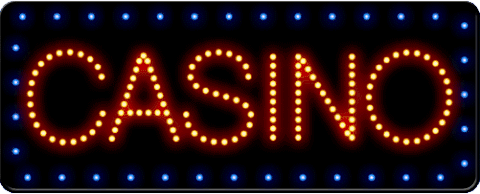In a casino on a Sunday, 90% of the visitors lost \$100 each, 9% of the visitors lost \$1,000 each, the rest won \$10,000 each. If the profit at the casino was \$80,000 for the day, how many people visited the casino?
Have you tried 22 tonight? I said 22.
ksdjdjJoined: Oct 20, 2013
• Posts: 1707
Thanks for this post from:June 25th, 2020 at 12:04:47 AM permalink
Quote: Gialmere

I get CP's numbers but, as you guys point out, it's not easy posting easy math problems.

So let me try...In a casino on a Sunday, 90% of the visitors lost \$100 each, 9% of the visitors lost \$1,000 each, the rest won \$10,000 each. If the profit at the casino was \$80,000 for the day, how many people visited the casino?

1000 people visited the casino.

"Working out" :
On that Sunday , the 'expected value' of one visitor to the casino was: (\$100 x 90%) + (\$1,000 x 9%) + (-\$10,000 x 1%) =
\$90 + \$90 - \$100 = \$80

"Profit for the day" / " 'expected value' of one visitor" = "the number of people that visited the casino (on that day)"

= \$80,000 / \$80 = 1000 people
GialmereJoined: Nov 26, 2018
• Posts: 2812
June 25th, 2020 at 6:00:28 AM permalink
Quote: ksdjdj

1000 people visited the casino.

"Working out" :
On that Sunday , the 'expected value' of one visitor to the casino was: (\$100 x 90%) + (\$1,000 x 9%) + (-\$10,000 x 1%) =
\$90 + \$90 - \$100 = \$80

"Profit for the day" / " 'expected value' of one visitor" = "the number of people that visited the casino (on that day)"

= \$80,000 / \$80 = 1000 people

Correct!
-------------------------

Q: How do you make a million dollars gambling?

Q: How do you get a professional poker player off your porch?
A: Pay him for the pizza.
Have you tried 22 tonight? I said 22.
gordonm888Joined: Feb 18, 2015
• Posts: 4818
June 25th, 2020 at 5:59:08 PM permalink
"Q: How do you get a professional poker player off your porch?
A: Pay him for the pizza."

This has always been one of my favorite gambling jokes. There is a sad truth to it.
So many better men, a few of them friends, are dead. And a thousand thousand slimy things live on, and so do I.
GialmereJoined: Nov 26, 2018
• Posts: 2812
June 27th, 2020 at 6:08:12 AM permalink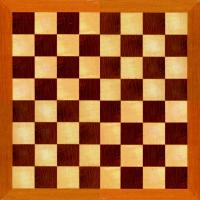And now a classic puzzle...

How many squares are there on a chessboard?
Have you tried 22 tonight? I said 22.
charliepatrickJoined: Jun 17, 2011
• Posts: 2937
Thanks for this post from:June 27th, 2020 at 7:05:10 AM permalink
There are 8^2 squares of size 1, 7^2 squares of size 2 (e.g. consider where their SW corner would be)... 1^2 of size 1.
Sum of the first n squares is n (n+1) (2n+1) / 6.
Using n=8 >gives 8 * 9 * 17 / 6 = 204.
unJonJoined: Jul 1, 2018
• Posts: 4352
June 27th, 2020 at 7:21:32 AM permalink
Quote: charliepatrick

There are 8^2 squares of size 1, 7^2 squares of size 2 (e.g. consider where their SW corner would be)... 1^2 of size 1.
Sum of the first n squares is n (n+1) (2n+1) / 6.
Using n=8 >gives 8 * 9 * 17 / 6 = 204.

But plus one more for the border.
The race is not always to the swift, nor the battle to the strong; but that is the way to bet.
GialmereJoined: Nov 26, 2018
• Posts: 2812
June 27th, 2020 at 8:19:27 AM permalink
Quote: charliepatrick

There are 8^2 squares of size 1, 7^2 squares of size 2 (e.g. consider where their SW corner would be)... 1^2 of size 1.
Sum of the first n squares is n (n+1) (2n+1) / 6.
Using n=8 >gives 8 * 9 * 17 / 6 = 204.

Correct
----------------------------

I like to play chess with old men in the park, although it's hard to find 32 of them.
Have you tried 22 tonight? I said 22.
GialmereJoined: Nov 26, 2018
• Posts: 2812
June 29th, 2020 at 8:14:02 AM permalink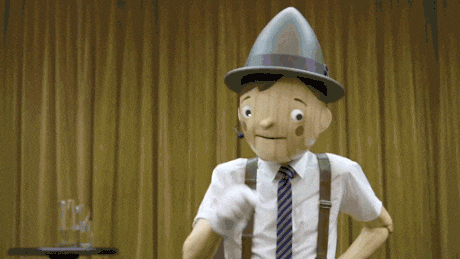When Pinocchio lies, his nose gets twice as long. When he tells the truth, his nose gets 1 cm shorter.

His nose was 1 cm long in the morning, and it is 100 cm long in the evening.

What is the least possible number of times he opened his mouth today?
Have you tried 22 tonight? I said 22.
ThatDonGuyJoined: Jun 22, 2011
• Posts: 5956
Thanks for this post from:June 29th, 2020 at 8:37:52 AM permalink
Quote: Gialmere

When Pinocchio lies, his nose gets twice as long. When he tells the truth, his nose gets 1 cm shorter.

His nose was 1 cm long in the morning, and it is 100 cm long in the evening.

What is the least possible number of times he opened his mouth today?

10 times, in this order:

Lie (2 cm), lie (4), lie (8), truth (7), lie (14), truth (13), lie (26), truth (25), lie (50), lie (100)

GialmereJoined: Nov 26, 2018
• Posts: 2812
June 29th, 2020 at 8:54:07 AM permalink
Quote: ThatDonGuy

10 times, in this order:

Lie (2 cm), lie (4), lie (8), truth (7), lie (14), truth (13), lie (26), truth (25), lie (50), lie (100)

Correct
--------------------------------

Voldemort: So I need to lie?

Pinocchio: Yup!
Have you tried 22 tonight? I said 22.
GialmereJoined: Nov 26, 2018
• Posts: 2812
July 1st, 2020 at 8:15:02 AM permalink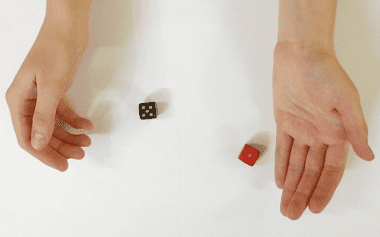After your earlier trials, you've come up with an idea for a casino dice game.

The player rolls one black die and one red die. If the black die gives an odd number, the player's overall score is zero. If the red die gives an even number, the player rolls it again and again until it is odd. The player's score is the sum of the two numbers, except for the previously mentioned case.

What is the average player score for this game?
Have you tried 22 tonight? I said 22.
unJonJoined: Jul 1, 2018
• Posts: 4352
Thanks for this post from:July 1st, 2020 at 9:44:03 AM permalink
Quote: GialmereAfter your earlier trials, you've come up with an idea for a casino dice game.

The player rolls one black die and one red die. If the black die gives an odd number, the player's overall score is zero. If the red die gives an even number, the player rolls it again and again until it is odd. The player's score is the sum of the two numbers, except for the previously mentioned case.

What is the average player score for this game?

I�m assuming that if the black die is even and the red die is even, then only the red die and not the black die is rolled again. If that�s right I get:

3.5
The race is not always to the swift, nor the battle to the strong; but that is the way to bet.
GialmereJoined: Nov 26, 2018
• Posts: 2812
July 1st, 2020 at 10:08:35 AM permalink
Quote: unJon

I�m assuming that if the black die is even and the red die is even, then only the red die and not the black die is rolled again. If that�s right I get:

3.5

Correct

[A player would probably need to score at least 5 points to be in the money.]
--------------------------

At a board game shop...

Customer: I want a dice.

Clerk: The correct term is 'die'.

Customer: I want 2 die.

Clerk: Plural is dice, alone it's die.

Customer: I want 2 die alone.
Have you tried 22 tonight? I said 22.
IAchance5Joined: May 28, 2010
• Posts: 167
Thanks for this post from:July 1st, 2020 at 10:39:52 AM permalink
Quote: GialmereAfter your earlier trials, you've come up with an idea for a casino dice game.

The player rolls one black die and one red die. If the black die gives an odd number, the player's overall score is zero. If the red die gives an even number, the player rolls it again and again until it is odd. The player's score is the sum of the two numbers, except for the previously mentioned case.

What is the average player score for this game?

So help me out on this....I tried to set it up like a typical dice throwing outcome chart, and then form a geometric series.

Pair of dice throw once (black die - y axis and red die - x axis) with sum outcomes:

1 2 3 4 5 6
1 0 0 0 0 0 0
2 3 4 5 6 7 8
3 0 0 0 0 0 0
4 5 6 7 8 9 10
5 0 0 0 0 0 0
6 7 8 9 10 11 12

The average of these outcomes = 3.75, so then I did a geometric series where a=3.75 and r = .5 (for 50% chance of rolling an even number on red die). this would give me 3.75/(1-.5) which comes to 7.5.

Maybe I assumed that both dice would continually be rolled over again until an odd number on the red? I'm also on my 2nd beer already though (took a long 4th of July weekend).....!

GialmereJoined: Nov 26, 2018
• Posts: 2812
July 1st, 2020 at 11:38:49 AM permalink
There are six possible scores: 0, 3, 5, 7, 9, and 11.

The probability of a 0 is 3/6 = 1/2.

If it is not 0, there are three options for black: 2, 4 and 6 and there are three options for red: 1, 3 and 5.

There are nine possible sums:
2 + 1 = 3
2 + 3 = 5
2 + 5 = 7
4 + 1 = 5
4 + 3 = 7
4 + 5 = 9
6 + 1 = 7
6 + 3 = 9
6 + 5 = 11

So the average score is...

0.5 x 0 + 0.5 x (3 + 5 + 7 + 5 +7 + 9 + 7 + 9 + 11) / 9 =

0.5 x 63 / 9 = 3.5
Have you tried 22 tonight? I said 22.
charliepatrickJoined: Jun 17, 2011
• Posts: 2937
Thanks for this post from:July 1st, 2020 at 11:44:20 AM permalink
Quote: Gialmere

...The player rolls one black die and one red die. If the black die gives an odd number, the player's overall score is zero. If the red die gives an even number, the player rolls it again and again until it is odd. The player's score is the sum of the two numbers, except for the previously mentioned case....

(i) Half the time the score is zero.
(ii) The other half the Black die is 2 4 or 6 (so has an average of 4), the White die will eventually be 1 3 or 5 (so has an average of 3); so the average total of a non-zero score is 7.
(iii) The average of 0 and 7 is 3.5; so that is the average score.
GialmereJoined: Nov 26, 2018
• Posts: 2812
July 1st, 2020 at 11:56:19 AM permalink
And, since I'm bored at work...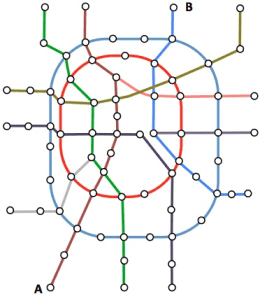The picture shows a subway map.

If it takes 3 minutes to travel from one station to the next station and 3 minutes to change lines, what is the minimum time required to go from A to B?
Have you tried 22 tonight? I said 22.
charliepatrickJoined: Jun 17, 2011
• Posts: 2937
Thanks for this post from:July 1st, 2020 at 12:56:35 PM permalink
Not easy to figure it out but here goes...
The method used by sat navs etc is to work out from either end, totalling the time to get to each place and see where the best route is. Using this I get various routes at 45 minutes, but the best I found was 42 minutes.

For simplicitiy my workings and the picture here assumed everything was 1 minute, so multiply the figures by 3. It's slightly confusing since you can get to station South of B in 39 minutes by various routes, but only the one where you're already on the blue line will get you to B in 42.unJonJoined: Jul 1, 2018
• Posts: 4352
July 1st, 2020 at 1:02:32 PM permalink
Quote: Gialmere

And, since I'm bored at work...The picture shows a subway map.

If it takes 3 minutes to travel from one station to the next station and 3 minutes to change lines, what is the minimum time required to go from A to B?

I�d be interested in knowing if there�s a non brute force way to do it. I just did the brute force method.
The race is not always to the swift, nor the battle to the strong; but that is the way to bet.
charliepatrickJoined: Jun 17, 2011
• Posts: 2937
July 1st, 2020 at 1:35:10 PM permalink
Quote: unJon

...I�d be interested in knowing if there�s a non brute force way to do it. I just did the brute force method.

I couldn't see a simpler way - and even now I've found a different route with the same time!
GialmereJoined: Nov 26, 2018
• Posts: 2812
July 1st, 2020 at 1:38:08 PM permalink
Quote: charliepatrick

Not easy to figure it out but here goes...

The method used by sat navs etc is to work out from either end, totalling the time to get to each place and see where the best route is. Using this I get various routes at 45 minutes, but the best I found was 42 minutes.

For simplicitiy my workings and the picture here assumed everything was 1 minute, so multiply the figures by 3. It's slightly confusing since you can get to station South of B in 39 minutes by various routes, but only the one where you're already on the blue line will get you to B in 42.Correct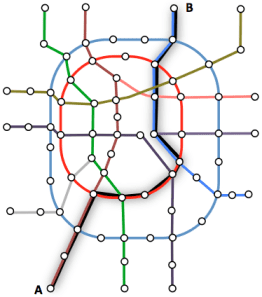A nice change of pace question.

--------------------------------

I was trying to make a funny subway pun but the joke got derailed.
Have you tried 22 tonight? I said 22.
ThatDonGuyJoined: Jun 22, 2011
• Posts: 5956
July 2nd, 2020 at 7:55:31 AM permalink
Here's a new one:

A school's volleyball team has N people on its roster, and its basketball team has N + 1 people on its roster.

The volleyball coach asked the school's computer boffins to come up with a list of all of the possible starting lineups of 6 players for the volleyball team.

The basketball coach thought this was a good idea, and asked for a list of all of the possible starting lineups of 5 players for the basketball team.

Both lists ended up being the same length (i.e. they both had the same number of starting lineups).

What is N?
rsactuaryJoined: Sep 6, 2014
• Posts: 2297
July 2nd, 2020 at 8:05:11 AM permalink
N=14. Tried it by formula, but made an error somewhere, ended up using brute force.

Figured out my error and proved formulaicly.

(n-4)(n-5)=6(n+1)

n=1 or 14 --> n cannot equal 1
ThatDonGuyJoined: Jun 22, 2011
• Posts: 5956
July 2nd, 2020 at 8:15:29 AM permalink
Quote: rsactuary

N=14. Tried it by formula, but made an error somewhere, ended up using brute force.

Figured out my error and proved formulaicly.

(n-4)(n-5)=6(n+1)

n=1 or 14 --> n cannot equal 1

That is correct...but in future, post answers in spoiler blocks.

As usual, (n)C(k) is how I write Combin(n,k)

There are (N)C(6) = N (N-1) (N-2) (N-3) (N-4) (N-5) / 720 volleyball lineups,
and (N+1)C(5) = (N+1) N (N-1) (N-2) (N-3) / 120 basketball lineups,
N (N-1) (N-2) (N-3) (N-4) (N-5) / 720 = (N+1) N (N-1) (N-2) (N-3) / 120
N (N-1) (N-2) (N-3) (N-4) (N-5) / 720 = 6 (N+1) N (N-1) (N-2) (N-3) / 720
Divide both sides by N (N-1) (N-2) (N-3) / 720:
(N-4) (N-5) = 6 (N+1)
N2 - 9 N + 20 = 6 N + 6
N2 - 15 N + 14 = 0
(N - 14) (N - 1) = 0
N >= 6 as otherwise there would not be enough players for any volleyball teams, so N = 14; there are 3003 possible lineups for each team

GialmereJoined: Nov 26, 2018
• Posts: 2812
July 2nd, 2020 at 7:31:13 PM permalinkA superstitious man is frightened by the number 13. He wants to set the four-digit passcode on his phone so that it does not have a digit 1 (if used) immediately followed by a digit 3 (if used) anywhere in the sequence. How many passcodes remain available to him?
Have you tried 22 tonight? I said 22.
unJonJoined: Jul 1, 2018
• Posts: 4352
Thanks for this post from:July 2nd, 2020 at 8:41:51 PM permalink
Quote: GialmereA superstitious man is frightened by the number 13. He wants to set the four-digit passcode on his phone so that it does not have a digit 1 (if used) immediately followed by a digit 3 (if used) anywhere in the sequence. How many passcodes remain available to him?

I think this is just:

9,700. Missing 100 combos that start 13XX and 100 that are X13X and 100 that are XX13

I mean 9,701 so you don�t double count 1313
The race is not always to the swift, nor the battle to the strong; but that is the way to bet.
GialmereJoined: Nov 26, 2018
• Posts: 2812
July 2nd, 2020 at 11:58:05 PM permalink
Quote: unJon

I think this is just:

9,700. Missing 100 combos that start 13XX and 100 that are X13X and 100 that are XX13

I mean 9,701 so you don�t double count 1313

Correct!

[The double was a twist.]
---------------------------

I think it's bad luck to believe in superstition.
Have you tried 22 tonight? I said 22.
GialmereJoined: Nov 26, 2018
• Posts: 2812
July 3rd, 2020 at 2:05:39 PM permalinkThere are three runners X, Y and Z. Each runs with a different uniform speed in a 1000 meters race.

If X gives Y a start of 50 meters, they will finish the race at the same time.

If X gives Z a start of 69 meters, they will finish the race at the same time.

Suppose Y and Z are in a race. How much of a start should Y give to Z so they would finish the race at the same time?
Have you tried 22 tonight? I said 22.
IAchance5Joined: May 28, 2010
• Posts: 167
Thanks for this post from:July 3rd, 2020 at 7:34:52 PM permalink
Quote: GialmereThere are three runners X, Y and Z. Each runs with a different uniform speed in a 1000 meters race.

If X gives Y a start of 50 meters, they will finish the race at the same time.

If X gives Z a start of 69 meters, they will finish the race at the same time.

Suppose Y and Z are in a race. How much of a start should Y give to Z so they would finish the race at the same time?

Hopefully this spoiler tag works....

So I think this is a distance problem, which X runs 1000m at the same time that Y runs 950m and Z runs 931m....which means that the rate of Y is .95 of X (950/1000) and Z is .931 of X (931/1000).

For the second race (Y & Z), I tried to determined the same thing as above but just comparing Y to Z:

{Y rate} (1/.95).95x = {Z rate} .931x(1/.95), which means that the rate of Z is .98 of Y.

Assuming that the race between Y and Z is also 1000m, then there should be a 20m difference between Y and Z.....hopefully I thought about this correctly but might not have explained it right.....
ThatDonGuyJoined: Jun 22, 2011
• Posts: 5956
Thanks for this post from:July 3rd, 2020 at 7:47:34 PM permalink
Quote: IAchance5

Quote: GialmereThere are three runners X, Y and Z. Each runs with a different uniform speed in a 1000 meters race.

If X gives Y a start of 50 meters, they will finish the race at the same time.

If X gives Z a start of 69 meters, they will finish the race at the same time.

Suppose Y and Z are in a race. How much of a start should Y give to Z so they would finish the race at the same time?

Hopefully this spoiler tag works....

So I think this is a distance problem, which X runs 1000m at the same time that Y runs 950m and Z runs 931m....which means that the rate of Y is .95 of X (950/1000) and Z is .931 of X (931/1000).

For the second race (Y & Z), I tried to determined the same thing as above but just comparing Y to Z:

{Y rate} (1/.95).95x = {Z rate} .931x(1/.95), which means that the rate of Z is .98 of Y.

Assuming that the race between Y and Z is also 1000m, then there should be a 20m difference between Y and Z.....hopefully I thought about this correctly but might not have explained it right.....

That is what I get, too

Let x, y, and z be the distance X, Y, and Z run in one second
X / 1000 = Y / 950 = Z / 931

Y / 1000 = Y / 950 * 950 / 1000 = Z / 931 * 19 / 20 = Z / 980

Y can run 1000m in the time it takes Z to run 980m, so Z should get a 20m head start

GialmereJoined: Nov 26, 2018
• Posts: 2812
July 3rd, 2020 at 9:31:38 PM permalink
Quote: IAchance5

Hopefully this spoiler tag works....

So I think this is a distance problem, which X runs 1000m at the same time that Y runs 950m and Z runs 931m....which means that the rate of Y is .95 of X (950/1000) and Z is .931 of X (931/1000).

For the second race (Y & Z), I tried to determined the same thing as above but just comparing Y to Z:

{Y rate} (1/.95).95x = {Z rate} .931x(1/.95), which means that the rate of Z is .98 of Y.

Assuming that the race between Y and Z is also 1000m, then there should be a 20m difference between Y and Z.....hopefully I thought about this correctly but might not have explained it right.....

Quote: ThatDonGuy

That is what I get, too

Let x, y, and z be the distance X, Y, and Z run in one second
X / 1000 = Y / 950 = Z / 931

Y / 1000 = Y / 950 * 950 / 1000 = Z / 931 * 19 / 20 = Z / 980

Y can run 1000m in the time it takes Z to run 980m, so Z should get a 20m head start

Correct!

Very observant as the question tries to trick you into a knee-jerk calculation based on a distance of only 950 meters.

--------------------

I�m like a cross between a marathon runner and a sprinter.

I can jog short distances.
Have you tried 22 tonight? I said 22.
GialmereJoined: Nov 26, 2018
• Posts: 2812
July 4th, 2020 at 7:44:38 AM permalink
Happy 4th of July!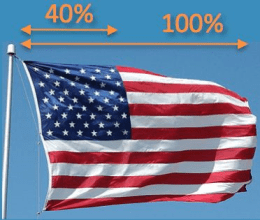Using the information in the picture above, what percentage of Old Glory is red?
Have you tried 22 tonight? I said 22.
Ace2Joined: Oct 2, 2017
• Posts: 2671
July 4th, 2020 at 8:13:32 AM permalink
342/845

7/13 * 7/13 * 3/5 + 6/13 * 1/2 * 1/1
Last edited by: Ace2 on Jul 4, 2020
It�s all about making that GTA
Gabes22Joined: Jul 19, 2011
• Posts: 1427
Thanks for this post from:July 4th, 2020 at 8:23:06 AM permalink
I get 27/65 or 41.54%

Assuming the full length stripes are 1/13 the area you have 3/13 below the star box in red. And then 4 stripes that are 3/5 the area or 60% the area of the 3 full length red stripes

3/5 × 4/13 = 12/65
3/13 × 5/5 = 15/65
12/65 + 15/65 = 27/65
Last edited by: Gabes22 on Jul 4, 2020
A flute with no holes is not a flute, a donut with no holes is a danish
charliepatrickJoined: Jun 17, 2011
• Posts: 2937
Thanks for this post from:July 4th, 2020 at 9:36:14 AM permalink
Quote: Gabes22

I get 27/65 or 41.54%...

I agree. Assume the flag is 10" wide and 13" deep (Yes I know it can't be, mathematically go with it!) then the total area is 130 sq". Each red stripe is 1" tall, three are 10" long and four are 6" long = 30+24 = 54 sq". So ratio is 54/130 = 27/65.

All the sizes are given here ... https://www.ushistory.org/betsy/flagetiq3.html
GialmereJoined: Nov 26, 2018
• Posts: 2812
July 4th, 2020 at 4:48:52 PM permalink
Quote: Gabes22

I get 27/65 or 41.54%

Assuming the full length stripes are 1/13 the area you have 3/13 below the star box in red. And then 4 stripes that are 3/5 the area or 60% the area of the 3 full length red stripes

3/5 × 4/13 = 12/65
3/13 × 5/5 = 15/65
12/65 + 15/65 = 27/65

Quote: charliepatrick

I agree. Assume the flag is 10" wide and 13" deep (Yes I know it can't be, mathematically go with it!) then the total area is 130 sq". Each red stripe is 1" tall, three are 10" long and four are 6" long = 30+24 = 54 sq". So ratio is 54/130 = 27/65.

All the sizes are given here ... https://www.ushistory.org/betsy/flagetiq3.html

Correct!

Without the blue Union canton: the red area is 7 stripes of 13.

The blue canton decreases the red area by 4 x 0.4 stripes = 1.6 stripes.

The red area is (7 - 1.6) / 13 = 5.4 / 13 = 27/65 = 0.4153 = 41.5% or 42%

----------------------------

The United States is a free country where every man can do as his wife pleases.
Have you tried 22 tonight? I said 22.
GialmereJoined: Nov 26, 2018
• Posts: 2812
July 5th, 2020 at 11:06:44 AM permalinkI toss a coin onto a chessboard. The diameter of the coin is half the side length of one of the small squares.

What is the probability that the coin touches both light and dark colors?

(I don't take into account cases when the coin touches the edge of the board.)
Have you tried 22 tonight? I said 22.
ThatDonGuyJoined: Jun 22, 2011
• Posts: 5956
July 5th, 2020 at 1:17:51 PM permalink
Quote: Gialmere

I toss a coin onto a chessboard. The diameter of the coin is half the side length of one of the small squares.

What is the probability that the coin touches both light and dark colors?

(I don't take into account cases when the coin touches the edge of the board.)

Let the length of a side of each square be 2; the board is 16 x 16, and the coin has diameter 1.

The center of the coin can land anywhere in the 14 x 14 square that is 1/2 from each side of the border of the board.
Er, that's a 15 x 15 square, isn't it?

The coin is entirely in a square if its center is within 1/2 of the center of the square; this has an area of 1/4.

The sum of the areas of the board where the coin does not touch both colors = 64 x 1/4 = 16, which means the total area where the center of the coin can land such that the coin does touch both is 196 - 64 = 132.
Make that 225 - 64 = 161

The probability of touching both colors = 132 / 196 = 33 / 49.
That's now 161 / 225

Last edited by: ThatDonGuy on Jul 5, 2020
charliepatrickJoined: Jun 17, 2011
• Posts: 2937
July 5th, 2020 at 1:32:47 PM permalink
Quote: Gialmere

...I toss a coin onto a chessboard. The diameter of the coin is half the side length of one of the small squares.
What is the probability that the coin touches both light and dark colors?
(I don't take into account cases when the coin touches the edge of the board.)

Lets assume each square is 4"x4" and the coin has a radius of 1" (i.e. has a width of 2"). The board is therefore 32"x32".

Ignoring the edge conditions (we'll look at those later) for the coin to be completely within any 4"x4" chess square, the coin's centre has to be within a square 2"x2". There are 64 chess squares so the total area of these 2"x2" squares is 64x4 = 256.

The coin cannot land over the edge, so it's centre cannot be within 1" of the edge of the board. As the board is 32"x32", removing a 1" border leaves 30"x30".

Area where the centre of the coin can land = 30x30 = 900.
Area where the coin is totally within a square = 256.
Area where the coin covers two colours is 900-256 = 644.

Probability of touching two colours = 644/900 = 161/255 ~= 63.14%.
GialmereJoined: Nov 26, 2018
• Posts: 2812
July 5th, 2020 at 5:44:22 PM permalink
Both incorrect. The solution is easier then you guys are making it (although you're both in the ballpark).

Remember the edge does not count. Either consider an edge result as "toss again" or that the board has infinite squares.
Have you tried 22 tonight? I said 22.
ThatDonGuyJoined: Jun 22, 2011
• Posts: 5956
Thanks for this post from:July 5th, 2020 at 5:59:31 PM permalink

Let the length of the side of each square be 2; the area of each square is 4, and the coin has radius 1/2.

The coin lands in a square if its center is more than one radius away from each edge; this is a 1 x 1 square, so it has area 1.

The probability of the coin touching both colors = the probability it does not land entirely within a square = 3/4.

I am pretty sure we both were counting "a coin landing on the edge is tossed again" when we came up with our earlier solutions. I certainly was; that is where 225 came in.

GialmereJoined: Nov 26, 2018
• Posts: 2812
July 5th, 2020 at 8:20:35 PM permalink
Quote: ThatDonGuy

Let the length of the side of each square be 2; the area of each square is 4, and the coin has radius 1/2.

The coin lands in a square if its center is more than one radius away from each edge; this is a 1 x 1 square, so it has area 1.

The probability of the coin touching both colors = the probability it does not land entirely within a square = 3/4.

I am pretty sure we both were counting "a coin landing on the edge is tossed again" when we came up with our earlier solutions. I certainly was; that is where 225 came in.

Correct!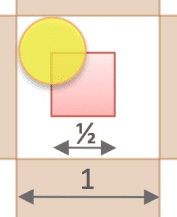--------------------------------

My laptop beat me at chess.

I then beat it at kickboxing.
Have you tried 22 tonight? I said 22.
GialmereJoined: Nov 26, 2018
• Posts: 2812
July 6th, 2020 at 9:21:25 AM permalinkFor a college play, all 20 theatre tickets for the first row were randomly distributed among 20 students. Both John and Jenny received a ticket.

What is the probability that John gets a seat next to his true love Jenny?
Have you tried 22 tonight? I said 22.
Ace2Joined: Oct 2, 2017
• Posts: 2671
Thanks for this post from:July 6th, 2020 at 9:50:37 AM permalink
Ten percent
It�s all about making that GTA
GialmereJoined: Nov 26, 2018
• Posts: 2812
July 6th, 2020 at 9:56:04 AM permalink
Quote: Ace2

Ten percent

Correct!

The end seats added some drama to that problem.
Have you tried 22 tonight? I said 22.
GialmereJoined: Nov 26, 2018
• Posts: 2812
July 6th, 2020 at 11:55:09 AM permalink
Another slow day at work so...Three sailors come across a pile of coconuts.

The fist sailor takes half of them plus half a coconut. The second sailor takes half of what is left, plus half a coconut. The third sailor takes half of what remains, plus half a coconut. Left over is exactly one coconut which they toss to a monkey.

How many coconuts were in the original pile?
Have you tried 22 tonight? I said 22.
Ace2Joined: Oct 2, 2017